# 为WordPress添加MathJax支持

#### MathJax是什么？

MathJax是一个开源的数学公式显示脚本。其最大的优势在于可以以基于文本的方式显示页面中的数学公式。而且支持Tex和LaTex、MathML、ASCIIMathML语言来书写公式，完美支持各大主流浏览器，拥有丰富的API接口。

#### 如何安装？

MathJax的安装十分简单，只需要一句代码就可以完成配置。

<script type="text/javascript" src="http://cdn.mathjax.org/mathjax/latest/MathJax.js?config=TeX-AMS-MML_HTMLorMML"></script>

Update:

function load_mathjax(){
wp_enqueue_script('mathjax', 'https://cdnjs.cloudflare.com/ajax/libs/mathjax/2.7.5/MathJax.js?config=TeX-AMS_CHTML,Safe', array(), '1.0', false);
}

add_action('wp_enqueue_scripts', 'load_mathjax');

#### 如何使用？\begin{aligned} \nabla \times \vec{\mathbf{B}} -\, \frac1c\, \frac{\partial\vec{\mathbf{E}}}{\partial t} & = \frac{4\pi}{c}\vec{\mathbf{j}} \\ \nabla \cdot \vec{\mathbf{E}} & = 4 \pi \rho \\ \nabla \times \vec{\mathbf{E}}\, +\, \frac1c\, \frac{\partial\vec{\mathbf{B}}}{\partial t} & = \vec{\mathbf{0}} \\ \nabla \cdot \vec{\mathbf{B}} & = 0 \end{aligned}

http://www.mathjax.org/

## 《为WordPress添加MathJax支持》上有11条评论

1.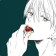梦月酱说道：

。。。。这个 不太需要

1.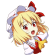hcl说道：

最近会写一点关于微积分的东西，这算是在做准备

1.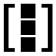大D说道：

这是要搞更高阶更牛的东西啊~~

1.hcl说道：

不敢当，只是写写自己的学习心得

1.大D说道：

那也不错，哈哈

2.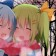酱爆灵梦说道：

数死早表示这么高级的东西伤不起

3.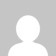鱼香肉丝说道：

为啥我加入这句话后 ，进入文章的预览模式之后 公式只看到了一眼，然后它就没了…

1.hcl说道：

本文中提到的方法仅适用于文章页面的显示，预览模式下可能并不会加载之间的脚本。

4.西域狂猪说道：

你好，请问楼主有写过矩阵吗？我不知道是语法写错了，还是 Mathjax 没生效，但是普通的等式可以加载出来的，而且我矩阵查了语句也没有问题，在CSDN上可以渲染。我使用过\begin{matrix}, \begin{bmatrix} 和 \begin{array}。感谢！

1.hcl说道：

经过我的测试，我这里的MathJax显示矩阵是没有问题的。显示出现问题可能要看你具体使用的加载方式和配置。文中使用的MathJax版本是2.7，现在最新的版本是3.0，两个版本之间的配置存在一定的差异，建议参考MathJax网站上的相关文档。本文仅仅提供了一个配置上的思路。如果在配置上确实有困难，直接使用WordPress插件仓库里的相关插件可能会更方便一些。

1.西域狂猪说道：

感谢，经过我测试发现是我的编辑器在渲染 markdown 时把我的换行的 \\ 去掉了，已经解决。感谢